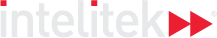### Course Overview

In the third course, the students become well-versed in many fundamental mathematical principles, including basic algebraic tenets. The first lessons begin with monomial and polynomial expressions and their various applications, leading to solving equations and the role of the law of order of operations.

HOURS OF INSTRUCTION: 15

### Course Outline

• Operations on Monomials and Polynomials
• Solving Simple Equations
• Algebraic Fractions
• Solving Linear Equations
• Basic Statistics
• Practical Applications